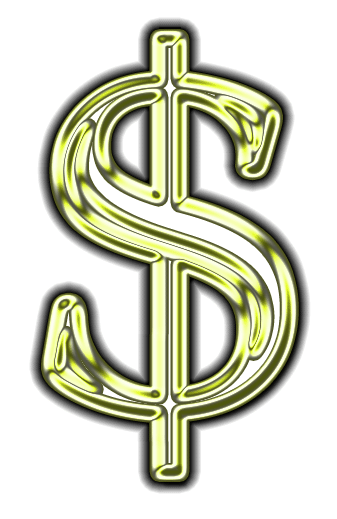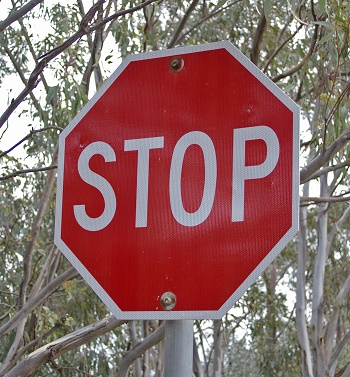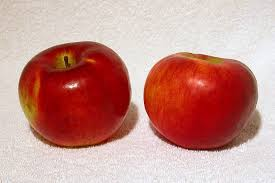What is a Digit in Math? - Definition & Examples

Instructor: Mark Boster
We use ten digits in the way we count. Sometimes we use one digit for to represent a number and sometimes we use more. In this lesson, you'll learn how many digits are in any given numeral, and recognize a digit when you see it. You will also begin learning about place value.

Symbols

You already know what this means:And you know this symbol:You also know what this is: 7.

That 7 is a special kind of symbol. It's called a digit. We have ten digits we use to make up all numerals. A numeral is a number written down. These digits are 0,1,2,3,4,5,6,7,8,and 9. That's it! You can make any numeral you want out of those ten digits.

Just like a red light means 'stop', a 7 means 'seven'. It's a single symbol that represents a numeral. Yes, just like this is a symbol to stop.You know that this is the number two.The numeral is 2, because there are two apples. You know there are five fingers on a hand - if you count the thumb! What numeral would that be? How about 5? Ding ding ding... That's right.

So What is a Number?

A number is the amount you are thinking, or the amount you see, like the apples or fingers above. As soon as you write it down it becomes a numeral. So...these are numerals: five, 5.

Counting Digits

This is the numeral: 51. These are the digits in that numeral: 5 and 1. The numeral has two digits because two symbols, or digits, make up the numeral 51. What about this numeral? 452. This numeral has 3 digits: 4, 5, and 2.

The numeral 15 has two digits; the two digits are 1 and 5.

This numeral has three digits: 102, a one (1), a zero (0), and a two (2). OK, lets try this one: 1595. I counted four: 1, 5, 9, and 5.

How about 150? Hmm... Three? 1, 5, and 0.

To unlock this lesson you must be a Study.com Member.

Register to view this lesson

Are you a student or a teacher?

See for yourself why 30 million people use Study.com

Become a Study.com member and start learning now.
Back
What teachers are saying about Study.com

Earning College Credit

Did you know… We have over 200 college courses that prepare you to earn credit by exam that is accepted by over 1,500 colleges and universities. You can test out of the first two years of college and save thousands off your degree. Anyone can earn credit-by-exam regardless of age or education level.